# Dirichlet product

## Definition

Suppose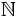is the set of natural numbers andis a commutative unital ring. Suppose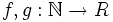are two functions. The Dirichlet product or Dirichlet convolution ofand, denoted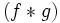, is defined as:.

The sum is over all positive integers dividing. Equivalently, it can be written as:.

Here, the summation is restricted to the cases where bothandare positive integers.

## Facts

### Ignoring the ring

Most of the functions we deal with are integer-valued. Note that there is a natural map from the integers to any commutative unital ring, and thus, any integer-valued function can be viewed as a function tofor any commutative unital ring. This makes most sense when the ring has characteristic zero, so that the map from integers to it is injective.

### Abelian monoid structure

The set of all functions fromtoforms a monoid with respect to the Dirichlet product:

• Dirichlet product is associative: We can see that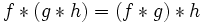, and both are equal to: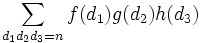.

• Dirichlet product is commutative: The fact that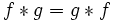is direct from the definition, and is based on the observation that the role of the divisorsand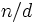can be switched.
• Identity element for Dirichlet product is indicator function for one: The identity element for the Dirichlet product is the function, defined as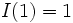, andfor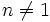.

### Abelian group structure

If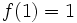(where theon the left is the natural number, and theon the right is the identity element of the ring), thenhas a multiplicative inverse with respect to the Dirichlet product. Moreover, this inverse also sendsto. The functions that sendto, in fact, form a group under the Dirichlet product. (More generally, we can look at all functions that sendto a unit).

An important subgroup of this group is the group of all multiplicative functions. A Dirichlet product of multiplicative functions is multiplicative, and the inverse of a multiplicative function is multiplicative. Further information: Multiplicative functions form a group under Dirichlet product

### Important functions

A complete list of commonly studied arithmetic functions is at:

Some particular important ones are:

• The identity element for Dirichlet product: Denoted, this is the indicator function for: it isatandelsewhere.
• The all ones function: This function sends everything to. This is denoted by. Note that although this is the identity for pointwise multiplication, it is not the identity for the Dirichlet product.
• The Mobius function: Denoted, this is the inverse of the all ones function with respect to the Dirichlet product.
• The identity function: This function sends every natural number to itself, now viewed as a ring element. This is denoted. Although this would be the identity for composition, it is not the identity for the Dirichlet product.